## Introduction

• Need simple models to estimate system performance in terms of signal delay and power dissipation.

• Issues include:
• Resistance, capacitance and inductance calculations.
• Delay estimations.
• Determination of conductor size for power and clock distribution.
• Power consumption.
• Charge sharing mechanisms.
• Design Margining.
• Reliability.
• Effects of scaling.

## Resistance Estimation

• The resistance of a uniform slab of conducting material may be expressed as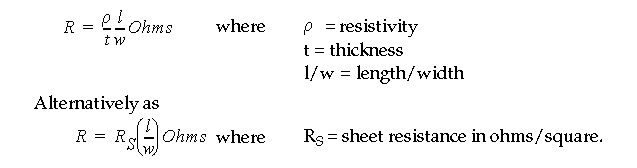• For example, in a layout editor, such as magic or virtuoso: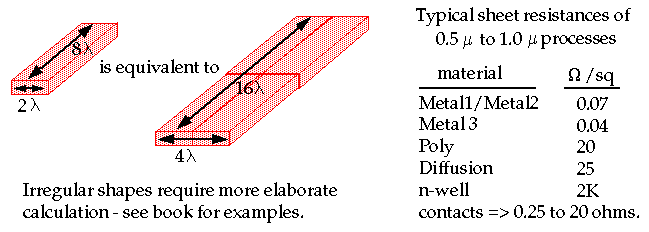## Resistance Estimation

• Channel resistance can be estimated in the linear region as:• A range of 1,000 to 30,000 ohms/square are possible for n-channel and p-channel devices.

• Typical betas for identically sized devices; n-dev: ~90, p-dev: ~30 microA/V 2 .

• Temperature changes both mu (mobility) and V t (threshold voltage) and, therefore channel resistance.

• Channel resistance increases with temperature, approximately +0.25% per degree C above 25 degrees.
• Metal and poly resistance change about 0.3% and well diffusions about 1% per degree C.

## Capacitance Estimation

• Switching speed of MOS systems strongly dependent:
• Parasitic capacitances associated with the MOS transistor.
• Interconnect capacitance of "wires".
• Resistance of transistors and wires.

• Total load capacitance on the output of a CMOS gate is sum of:
• Gate capacitance (of receiver logic gates downstream).
• Driver diffusion (source/drain) capacitance.
• Routing ( line ) capacitance of substrate and other wires.

• Let's consider approximations of each of these capacitances and subsequent approximations of delay based on these expressions.## Estimating Gate Capacitance:• The capacitance of a MOS transistor can be modeled using 5 capacitors.

• An approximation of gate capacitance (C gs , C gd and C gb ) is given as: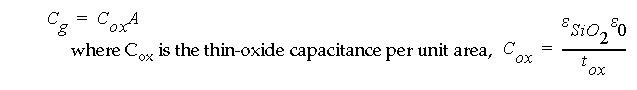## Estimating Gate Capacitance:

• For example, for thin-oxide thickness of 15 nm,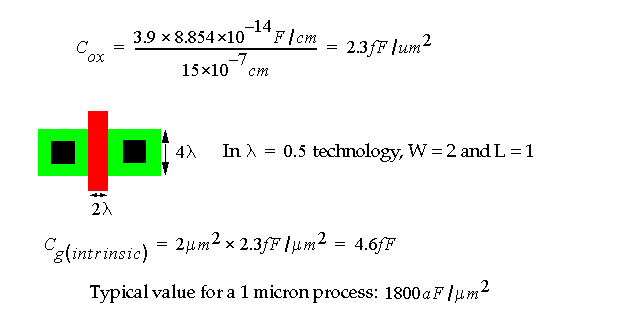• This is a conservative estimate of gate capacitance that does not include fringing fields (extrinsic) gate capacitance.

• Gate capacitance increases as the thin-oxide thins.

## Estimating Source/Drain Capacitance:

• An approximation ( lumped model ) of source/drain capacitance (C sb and C db ) is given as: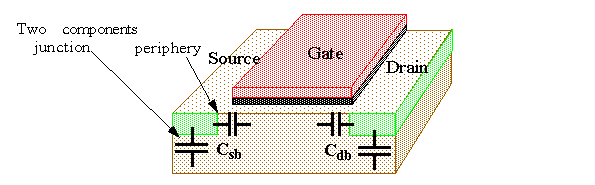• This model assumes a zero DC bias across the junction.

## Estimating Source/Drain Capacitance:

• For example: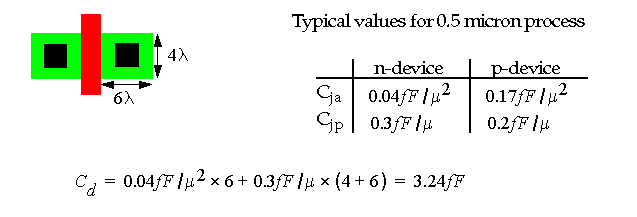## Estimating Routing Capacitance:

• Routing capacitance between metal and poly can be approximated using a parallel-plate model.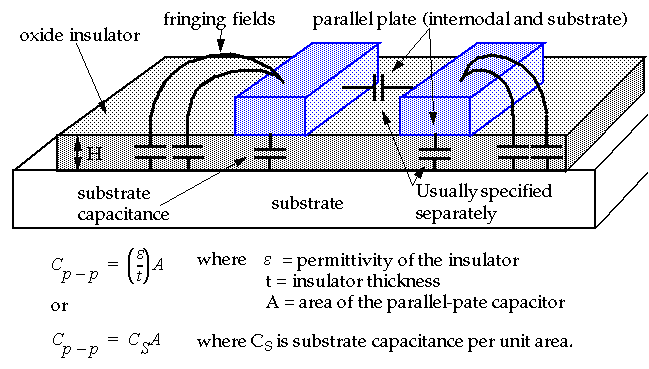• The effect of the fringing fields is to increase the effective area of the plates.

## Simple Gate Delay Model

• Appropriate if the wire delay is MUCH less than the gate delay, e.g.,• This expression derives from the expression for RC delay (we'll see this later).

• As an example, assuming gate delay is 200ps, what is the maximum length of a minimal-width metal wire (in 1.0um technology) that we can use without worrying about the RC delay of the wire itself?
• Assume Metal1 = 0.05 Ohms/square and 30 aF/um 2 .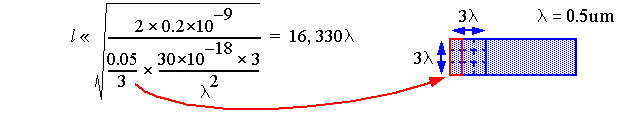• But this assumes there is no gate load capacitance.
• A conservative estimate is 5000 lambda (~16,330/3).
• In a 1.0um process, RC delay MUST be considered for any wire > 2.5mm.

## Simple Gate Delay Model

• But for now, let's consider "electrical nodes" for which we can ignore distributed RC effects.

• Our model and definitions: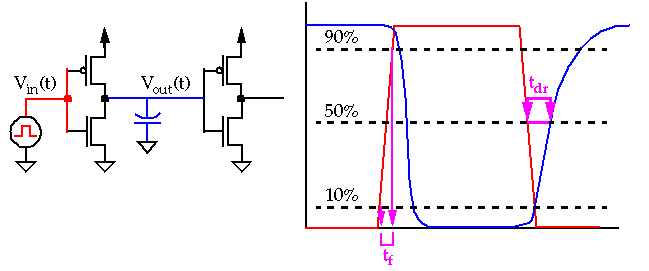• Fall/rise time, e.g. t f , computed between 10% and 90% of V DD.
• Propagation delay, t dr , computed at 50% points on input and output waveforms.

## Simple Gate Delay Model

• How do we model gate delay?
• Assume input is driven by a step waveform (unlike previous slide).

• Approximation for fall time: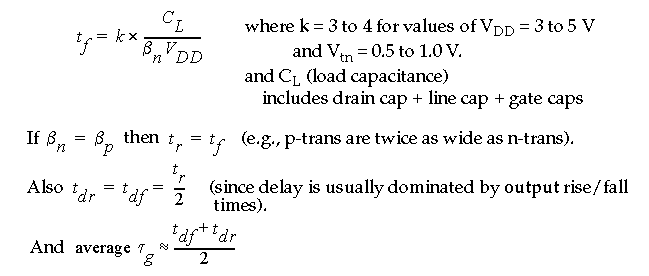• Note that the input waveform's finite slope will also effect this result -- adding a small amount of additional delay which is ignored here -- see text for details.

## Simple Gate Delay Model

• For example, let's compute the delay between G D and G R :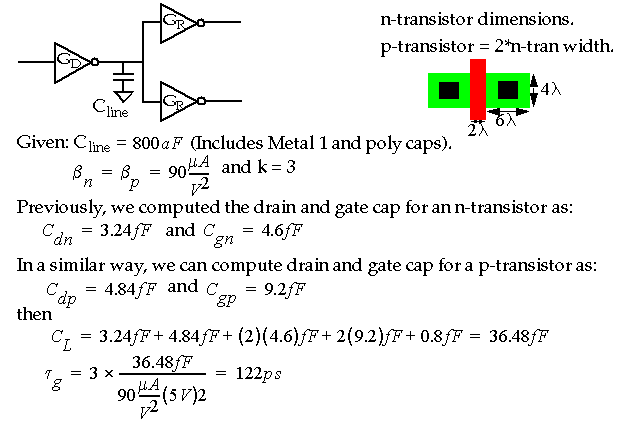## Distributed RC effects

• If the wire delay ~= gate delay, then we will have to use a different approximation consisting of three components: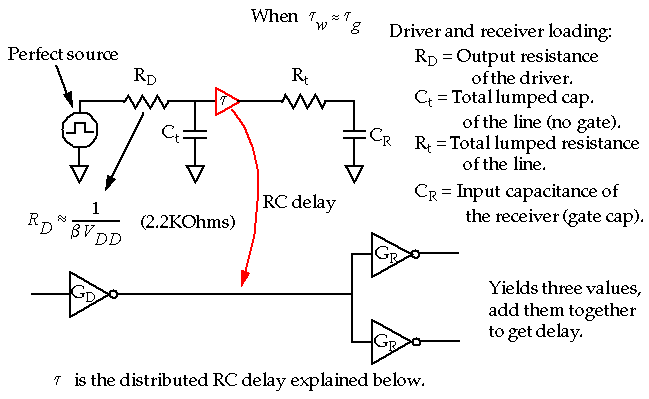## Distributed RC effects

• A wire can be represented in terms of several RC sections:• A discrete analysis of this circuit yields an approximate delay of:• As n becomes large (and the sections becomes small), this reduces to:• RC effect dominates for very long wires due to l 2 term, e.g., doubling the length of the wire, quadruples the delay.

## Distributed RC Effects

• For example, consider a long poly wire: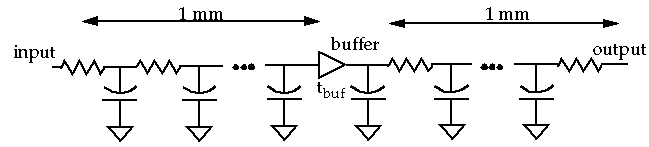• The buffer is one possible method of reducing the propagation delay.

• Assume r = 20 Ohms/micron and c = 0.4 fF/micron, then: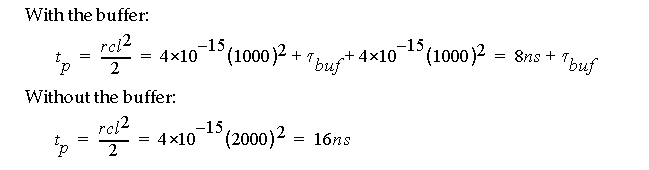• The buffer version is faster if its delay is less then 8ns. This is easily achieved.

## Distributed RC Effects

• When are distributed RC effects important to consider:
• Long wires with high resistance, e.g. poly wires.
• Long, heavily loaded clock lines.## Distributed RC Effects

• An example showing that reducing R at the expense of C helps a lot in some cases:
• Assume clock wire runs over 20mm and 50pF is distributed evenly along the line.
• Assume r = 0.05 Ohms/um.
• Then clock skew (delay to the end of the wire) is:• Solutions include adding a buffer, distributing the clock from the top center and/or widening the metal wire.

• For example, reducing l to 10mm and widening the clock wire to 20um:## Distributed RC Effects

• How does the distributed RC model differ from lumped model?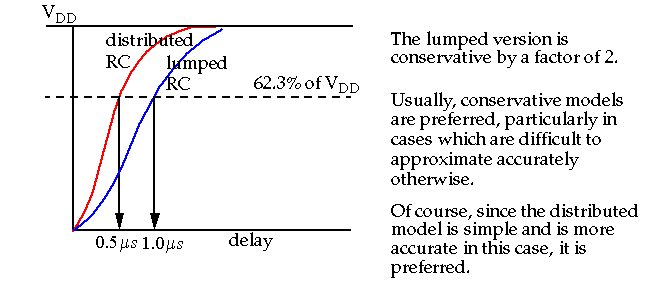• Note that these effects are completely ignored in the simple gate delay model.

• FYI: We estimate delay using RC time constants assuming that the time taken for a signal to reach 62.3% of its final value approximates the switching point of an inverter.

## Gate Delays

• Construct an equivalent inverter, e.g.,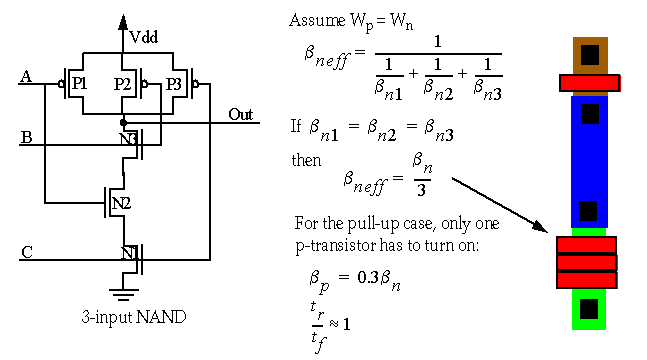• 3-input NANDs are closely balanced since n beta is about 3 times larger than p beta.